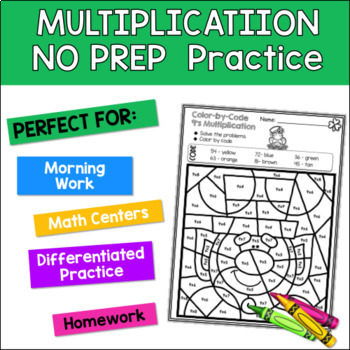DID YOU KNOW:
Seamlessly assign resources as digital activities

Learn how in 5 minutes with a tutorial resource. Try it Now

Learn More# Multiplication Color by Code | {2’s - 9's} {St. Patrick's Day}3rd - 5th, Homeschool
Subjects
Standards
Resource Type
Formats Included
• PDF
• Compatible withActivities
Pages
23 pages
Compatible with Easel Activities
Create an interactive version of this PDF students can complete on any device. Easel is free to use! Learn more.

#### Also included in

1. Need some NO-PREP, engaging seatwork for your students during holiday seasons? These color-by-code coloring pages will give your students that essential non-threatening practice they need for multiplication facts – and they will be engaged!This bundle contains 8 Holiday packs with 64 pages of engag
\$25.20
\$28.00
Save \$2.80
2. Are your students needing some engaging, effective, and NO PREP multiplication fact practice for your students?! Look no further! These color by Code Addition worksheets are perfect improving their math fact fluency and may used for:Early FinishersIndependent Practice SeatworkMath CentersReviewHomew
\$47.60
\$59.50
Save \$11.90

### Description

Need some no-prep, engaging seatwork for your students during some of your winter days? Your students will love these St. Patrick-themed color by code worksheets. They will be getting that essential, non-threatening practice they need for multiplication fact mastery – and they will be engaged!!!

Perfect for:
• Independent Practice Seatwork
• Math Centers
• Review
• Homework
• Morning Work
• RTI pull out

INCLUDED RESOURCES ★
★ 8 Color-by-Code:
• x 4
• x 5
• x 6
• x 7
• x 8
• x 9
• Mixed Practice 2’s – 5’s
• Mixed Practice 6’s – 10’s

------------------------------------------------------------------
------------------------------------------------------------------
Customer Tips:

♥ Click on the ★ above (by my picture) to follow my store.

♥ Leave feedback to earn credit points to save money on future products!
------------------------------------------------------------------
Pinterest
Instagram
Peggy @ Primary Flourish
------------------------------------------------------------------
Total Pages
23 pages
Included
Teaching Duration
N/A
Report this Resource to TpT
Reported resources will be reviewed by our team. Report this resource to let us know if this resource violates TpT’s content guidelines.

### Standards

to see state-specific standards (only available in the US).
Fluently multiply and divide within 100, using strategies such as the relationship between multiplication and division (e.g., knowing that 8 × 5 = 40, one knows 40 ÷ 5 = 8) or properties of operations. By the end of Grade 3, know from memory all products of two one-digit numbers.
Apply properties of operations as strategies to multiply and divide. Examples: If 6 × 4 = 24 is known, then 4 × 6 = 24 is also known. (Commutative property of multiplication.) 3 × 5 × 2 can be found by 3 × 5 = 15, then 15 × 2 = 30, or by 5 × 2 = 10, then 3 × 10 = 30. (Associative property of multiplication.) Knowing that 8 × 5 = 40 and 8 × 2 = 16, one can find 8 × 7 as 8 × (5 + 2) = (8 × 5) + (8 × 2) = 40 + 16 = 56. (Distributive property.)
Determine the unknown whole number in a multiplication or division equation relating three whole numbers. For example, determine the unknown number that makes the equation true in each of the equations 8 × ? = 48, 5 = __ ÷ 3, 6 × 6 = ?.
Use multiplication and division within 100 to solve word problems in situations involving equal groups, arrays, and measurement quantities, e.g., by using drawings and equations with a symbol for the unknown number to represent the problem.
Interpret products of whole numbers, e.g., interpret 5 × 7 as the total number of objects in 5 groups of 7 objects each. For example, describe a context in which a total number of objects can be expressed as 5 × 7.